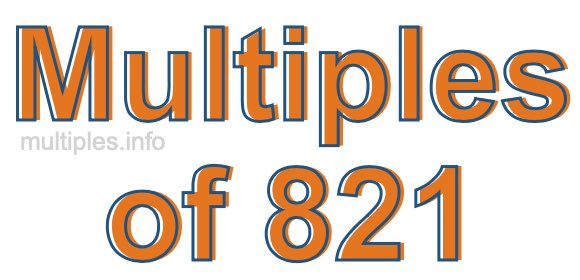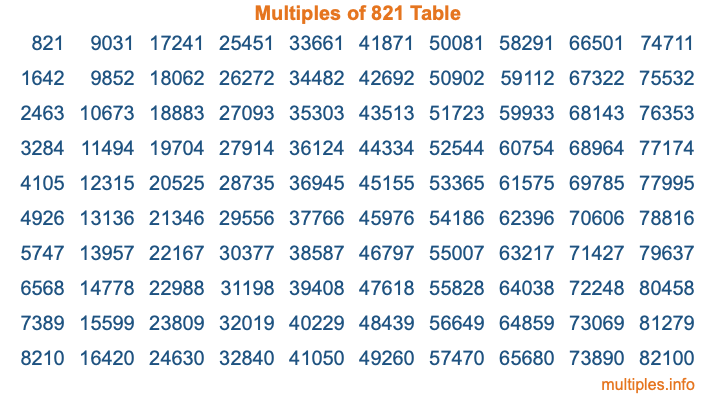Multiples of 821Welcome to the Multiples of 821 page. Here we will first teach you everything you will ever need to know about the multiples of 821, and then give you a study guide summary of everything we taught you to make sure you remember it all. Use this page to look up facts and learn information about the multiples of 821. This page will make you a multiples of eight hundred twenty-one expert!

Definition of Multiples of 821
Multiples of 821 are all the numbers that when divided by 821 equal an integer. Each of the multiples of 821 are called a multiple. A multiple of 821 is created by multiplying 821 by an integer.

Therefore, to create a list of multiples of 821, you start with 1 multiplied by 821, then 2 multiplied by 821, then 3 multiplied by 821, and so on for as long as you want. Thus, the list of the first five multiples of 821 is 821, 1642, 2463, 3284, and 4105. To see a larger list of multiples of 821, see the printable image of Multiples of 821 further down on this page. We also have a category where you can choose any nth multiple of 821.

Multiples of 821 Checker
The Multiples of 821 Checker below checks to see if any number of your choice is a multiple of 821. In other words, it checks to see if there is any number (integer) that when multiplied by 821 will equal your number. To do that, we divide your number by 821. If the the quotient is an integer, then your number is a multiple of 821.

Is  a multiple of 821?

Least Common Multiple of 821 and ...
A Least Common Multiple (LCM) is the lowest multiple that two or more numbers have in common. This is also called the smallest common multiple or lowest common multiple and is useful to know when you are adding our subtracting fractions. Enter one or more numbers below (821 is already entered) to find the LCM.

Check out our LCM Calculator if you need more details about the Least Common Multiple or if you need the LCM for different numbers for adding and subtraction fractions.

nth Multiple of 821
As we stated above, 821 is the first multiple of 821, 1642 is the second multiple of 821, 2463 is the third multiple of 821, and so on. Enter a number below to find the nth multiple of 821.

th multiple of 821

Multiples of 821 vs Factors of 821
821 is a multiple of 821 and a factor of 821, but that is where the similarities end. All postive multiples of 821 are 821 or greater than 821. All positive factors of 821 are 821 or less than 821.

Below is the beginning list of multiples of 821 and the factors of 821 so you can compare:

Multiples of 821: 821, 1642, 2463, 3284, 4105, etc.

Factors of 821: 1, 821

As you can see, the multiples of 821 are all the numbers that you can divide by 821 to get a whole number. The factors of 821, on the other hand, are all the whole numbers that you can multiply by another whole number to get 821.

It's also interesting to note that if a number (x) is a factor of 821, then 821 will also be a multiple of that number (x).

Multiples of 821 vs Divisors of 821
The divisors of 821 are all the integers that 821 can be divided by evenly. Below is a list of the divisors of 821.

Divisors of 821: 1, 821

The interesting thing to note here is that if you take any multiple of 821 and divide it by a divisor of 821, you will see that the quotient is an integer.

Multiples of 821 Table
Below is an image of the first 100 multiples of 821 in a table. The table is in chronological order, column by column. The first column has the first ten multiples of 821, the second column has the next ten multiples of 821, and so on.The Multiples of 821 Table is also referred to as the 821 Times Table or Times Table of 821. You are welcome to print out our table for your studies.

Negative Multiples of 821
Although not often discussed or needed in math, it is worth mentioning that you can make a list of negative multiples of 821 by multiplying 821 by -1, then by -2, then by -3, and so on, to get the following list of negative multiples of 821:

-821, -1642, -2463, -3284, -4105, etc.

Multiples of 821 Summary
Below is a summary of important Multiples of 821 facts that we have discussed on this page. To retain the knowledge on this page, we recommend that you read through the summary and explain to yourself or a study partner why they hold true.

There are an infinite number of multiples of 821.

A multiple of 821 divided by 821 will equal a whole number.

821 divided by a factor of 821 equals a divisor of 821.

The nth multiple of 821 is n times 821.

The largest factor of 821 is equal to the first positive multiple of 821.

821 is a multiple of every factor of 821.

821 is a multiple of 821.

A multiple of 821 divided by a divisor of 821 equals an integer.

821 divided by a divisor of 821 equals a factor of 821.

Any integer times 821 will equal a multiple of 821.

Multiples of a Number
Here you can get the multiples of another number, all with the same attention to detail as we did for multiples of 821 on this page.

Multiples of
Multiples of 822
Did you find our page about multiples of eight hundred twenty-one educational? Do you want more knowledge? Check out the multiples of the next number on our list!